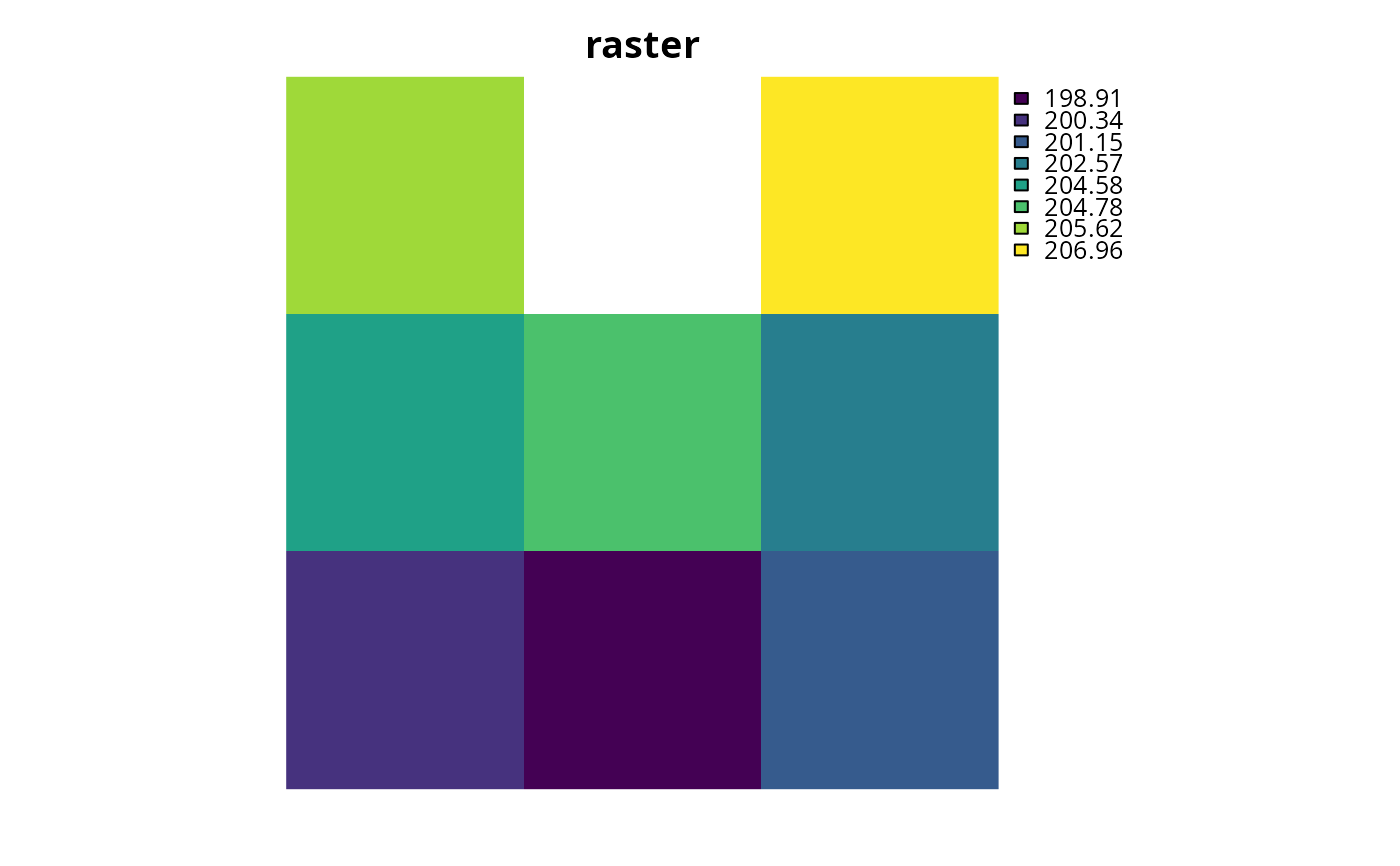Create a matrix showing which planning units are spatially adjacent to each other. Note that this also include planning units that overlap with each other too.

adjacency_matrix(x, ...)

# S3 method for Raster

# S3 method for SpatialPolygons

# S3 method for SpatialLines

# S3 method for SpatialPoints

# S3 method for sf

# S3 method for default
adjacency_matrix(x, ...)

## Arguments

x

Raster, SpatialPolygons, SpatialLines, or sf::sf() object representing planning units.

...

not used.

directions

integer If x is a Raster object, the number of directions in which cells should be considered adjacent: 4 (rook's case), 8 (queen's case), 16 (knight and one-cell queen moves), or "bishop" to for cells with one-cell diagonal moves.

## Value

dsCMatrix sparse symmetric matrix. Each row and column represents a planning unit. Cells values indicate if different planning units are adjacent to each other or not (using ones and zeros). To reduce computational burden, cells among the matrix diagonal are set to zero. Furthermore, if the argument to x is a Raster object, then cells with NAvalues are set to zero too.

## Details

Spatial processing is completed using sf::st_intersects() for Spatial and sf::sf() objects, and raster::adjacent() for Raster objects.

## Examples

# load data
data(sim_pu_raster, sim_pu_sf, sim_pu_lines)

# create adjacency matrix using raster data
## crop raster to 9 cells
r <- crop(sim_pu_raster, c(0, 0.3, 0, 0.3))

# create adjacency matrix using polygons (sf) data
## subset 9 polygons
ply <- sim_pu_sf[c(1:2, 10:12, 20:22), ]

# create adjacency matrix using lines (Spatial) data
## subset 9 lines
lns <- sim_pu_lines[c(1:2, 10:12, 20:22), ]

# plot data and the adjacency matrices
# \dontrun{
par(mfrow = c(4,2))

## plot raster and adjacency matrix
plot(r, main = "raster", axes = FALSE, box = FALSE)
plot(raster(as.matrix(am_raster)), main = "adjacency matrix", axes = FALSE,
box = FALSE)

## plot polygons (sf) and adjacency matrix
plot(r, main = "polygons (sf)", axes = FALSE, box = FALSE)
plot(raster(as.matrix(am_ply)), main = "adjacency matrix", axes = FALSE,
box = FALSE)

## plot lines (Spatial) and adjacency matrix
plot(r, main = "lines (Spatial)", axes = FALSE, box = FALSE)
plot(raster(as.matrix(am_lns)), main = "adjacency matrix", axes = FALSE,
box = FALSE)
# }